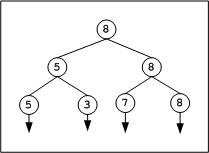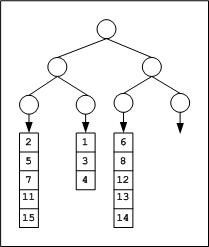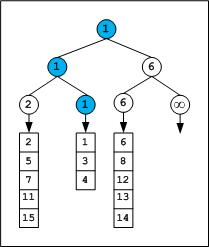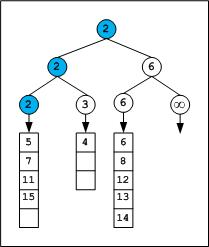# Tournament Tree (Winner Tree) and Binary Heap

• Difficulty Level : Hard
• Last Updated : 28 Sep, 2018

Given a team of N players. How many minimum games are required to find second best player?

We can use adversary arguments based on tournament tree (Binary Heap).

Attention reader! Don’t stop learning now. Get hold of all the important DSA concepts with the DSA Self Paced Course at a student-friendly price and become industry ready.  To complete your preparation from learning a language to DS Algo and many more,  please refer Complete Interview Preparation Course.

In case you wish to attend live classes with experts, please refer DSA Live Classes for Working Professionals and Competitive Programming Live for Students.

Tournament tree is a form of min (max) heap which is a complete binary tree. Every external node represents a player and internal node represents winner. In a tournament tree every internal node contains winner and every leaf node contains one player.

There will be N – 1 internal nodes in a binary tree with N leaf (external) nodes. For details see this post (put n = 2 in equation given in the post).

It is obvious that to select the best player among N players, (N – 1) players to be eliminated, i.e. we need minimum of (N – 1) games (comparisons). Mathematically we can prove it. In a binary tree I = E – 1, where I is number of internal nodes and E is number of external nodes. It means to find maximum or minimum element of an array, we need N – 1 (internal nodes) comparisons.

Second Best Player

The information explored during best player selection can be used to minimize the number of comparisons in tracing the next best players. For example, we can pick second best player in (N + log2N – 2) comparisons.

The following diagram displays a  tournament tree (winner tree) as a max heap. Note that the concept of loser tree is different.The above tree contains 4 leaf nodes that represent players and have 3 levels 0, 1 and 2. Initially 2 games are conducted at level 2, one between 5 and 3 and another one between 7 and 8. In the next move, one more game is conducted between 5 and 8 to conclude the final winner. Overall we need 3 comparisons. For second best player we need to trace the candidates participated with final winner, that leads to 7 as second best.

Median of Sorted Arrays

Tournament tree can effectively be used to find median of sorted arrays. Assume, given M sorted arrays of equal size L (for simplicity). We can attach all these sorted arrays to the tournament tree, one array per leaf. We need a tree of height CEIL (log2M) to have atleast M external nodes.

Consider an example. Given 3 (M = 3) sorted integer arrays of maximum size 5 elements.

```{ 2, 5, 7, 11, 15 } ---- Array1
{1, 3, 4} ---- Array2
{6, 8, 12, 13, 14} ---- Array3```

What should be the height of tournament tree? We need to construct a tournament tree of height log23 .= 1.585 = 2 rounded to next integer. A binary tree of height 2 will have 4 leaves to which we can attach the arrays as shown in the below figure.After the first tournament, the tree appears as below,We can observe that the winner is from Array2. Hence the next element from Array2 will dive-in and games will be played along the winner path of previous tournament.

Note that infinity is used as sentinel element. Based on data being hold in nodes we can select the sentinel character. For example we usually store the pointers in nodes rather than keys, so NULL can serve as sentinel. If any of the array exhausts we will fill the corresponding leaf and upcoming internal nodes with sentinel.

After the second tournament, the tree appears as below,The next winner is from Array1, so next element of Array1 array which is 5 will dive-in to the next round, and next tournament played along the path of 2.

The tournaments can be continued till we get median element which is (5+3+5)/2 = 7th element. Note that there are even better algorithms for finding median of union of sorted arrays, for details see the related links given below.

In general with M sorted lists of size L1, L2 … Lm requires time complexity of O((L1 + L2 + … + Lm) * logM) to merge all the arrays, and O(m*logM) time to find median, where m is median position.

Select smallest one million elements from one billion unsorted elements:

As a simple solution, we can sort the billion numbers and select first one million.

On a limited memory system sorting billion elements and picking the first one million seems to be impractical. We can use tournament tree approach. At any time only elements of tree to be in memory.

Split the large array (perhaps stored on disk) into smaller size arrays of size one million each (or even smaller that can be sorted by the machine). Sort these 1000 small size arrays and store them on disk as individual files. Construct a tournament tree which can have atleast 1000 leaf nodes (tree to be of height 10 since 29 < 1000 < 210, if the individual file size is even smaller we will need more leaf nodes). Every leaf node will have an engine that picks next element from the sorted file stored on disk. We can play the tournament tree game to extract first one million elements.

Total cost = sorting 1000 lists of one million each + tree construction + tournaments

Implementation
We need to build the tree in bottom-up manner. All the leaf nodes filled first. Start at the left extreme of tree and fill along the breadth (i.e. from 2k-1 to 2k – 1 where k is depth of tree) and play the game. After practicing with few examples it will be easy to write code. Implementation is discussed in below code

Second minimum element using minimum comparisons